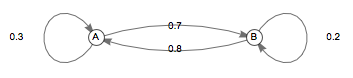# What is the Transition Matrix?

A Markov chain has first state A and second state B, and its transition probabilities for all time are given by the following graph:What is its transition matrix?

Note: The transition matrix is oriented such that the $k^\text{th}$ row represents the set of probabilities of transitioning from state $k$ to another state.

×